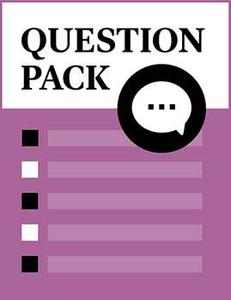Probability & Descriptive Statistics

Source: Sofia Open Content Initative

Student Price: FREE

An introductory statistics question pack from the Sofia Open Content Initiative.

Probability & Descriptive Statistics Q1

A sample of 56 employees was taken to determine average pulse rate. The data is in the table below. The mode(s) is/are $\rule{1cm}{0.15mm}$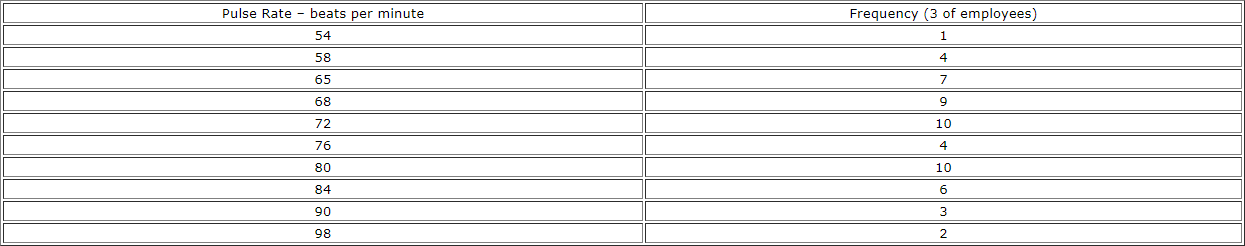A

74

B

72 and 80

C

68

D

the average of 72 and 80

Probability & Descriptive Statistics Q2

A sample of 56 employees was taken to determine average pulse rate. The data is in the table below. The variable is $\rule{1cm}{0.15mm}$A

the number of employees

B

all employees

C

the average pulse rate for the 56 employees

D

the pulse rate of one employee

Probability & Descriptive Statistics Q3

The following table shows the ages of 50 senior citizens who attend a local senior center. Find the IQR.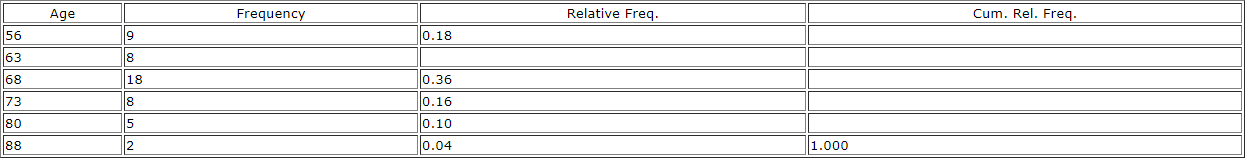A

0.5

B

17

C

10

D

Not enough information

Probability & Descriptive Statistics Q4

The following table shows the ages of 50 senior citizens who attend a local senior center. Which interval has the smallest percentage of data?A

The interval 55.5 to 63.5.

B

The interval 63.5 to 68.5.

C

The interval 68.5 to 73.5.

D

The interval 73.5 to 88.5.

Probability & Descriptive Statistics Q5

The following table shows the ages of 50 senior citizens who attend a local senior center. What percent of the ages are at most 68?A

36

B

70

C

30

D

66

Probability & Descriptive Statistics Q6

What can be said about a set of data when its standard deviation is zero?

A

If the data are ordered, they are very spread out from the mean.

B

All of the data appear with the same frequency.

C

If the data are ordered, they are very close to but different from the mean.

D

There is no mode.

Probability & Descriptive Statistics Q7

For the following data, which measure of central tendency would be the $\textbf{least}$ useful?

Data: 4, 8, 11, 11, 11, 27, 27, 27, 27, 27, 1000

A

mean

B

median

C

mode

D

standard deviation

Probability & Descriptive Statistics Q8

Sixty (60) college students were asked the number of units they are taking this quarter. The results are given in the graph below. The number of responses that were “12” OR “13” is approximately $\rule{1cm}{0.15mm}$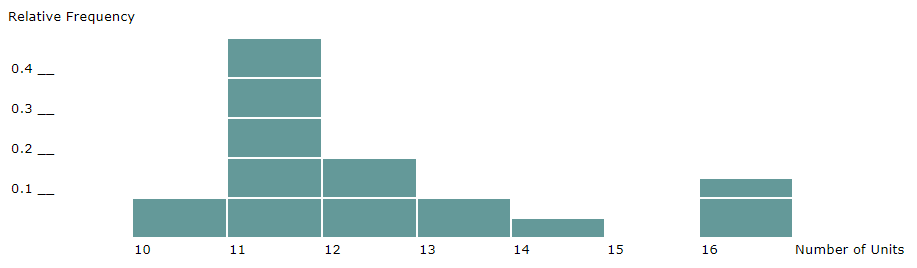A

18

B

0.3

C

25

D

Not enough information

Probability & Descriptive Statistics Q9

Sixty (60) college students were asked the number of units they are taking this quarter. The results are given in the graph below. The third quartile is $\rule{1cm}{0.15mm}$A

15

B

14

C

13

D

12

Probability & Descriptive Statistics Q10

The sample was taken by randomly selecting one student from the administration’s official list of students and then choosing every 100th. This is an example of what kind of sampling?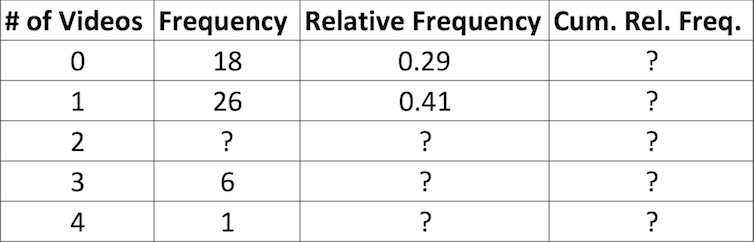A

cluster

B

systematic

C

stratified

D

convenience

Probability & Descriptive Statistics Q11

How should you classify data from the following question: What is your blood pressure?

A

qualitative

B

quantitative – discrete

C

quantitative - continuous

Probability & Descriptive Statistics Q12

How should you classify data from the following question: What is your favorite vacation place?

A

qualitative

B

quantitative – discrete

C

quantitative – continuous

Probability & Descriptive Statistics Q13

A study is done to determine the average amount of tuition all San Jose State undergraduate students pay per semester. A sample of 100 undergraduate San Jose State students is taken by dividing the students into freshmen, sophomore, junior, and senior years and selecting 25 students from each. Each student is asked how much tuition he/she paid for the Spring 2002 semester. The type of sampling and the parameter are:

A

cluster and the average amount of tuition all San Jose State students paid Spring 2002.

B

stratified and the average amount of tuition the 100 San Jose State students paid Spring 2002.

C

cluster and the average amount of tuition the 100 San Jose State students paid Spring 2002.

D

stratified and the average amount of tuition all San Jose State students paid Spring 2002.

Probability & Descriptive Statistics Q14

The following table classified eighty-two children by age and favorite meals at Mac Donald restaurant. Assume a child is randomly selected. Find the probability of being “7-12” years old AND preferring “chicken nuggets”.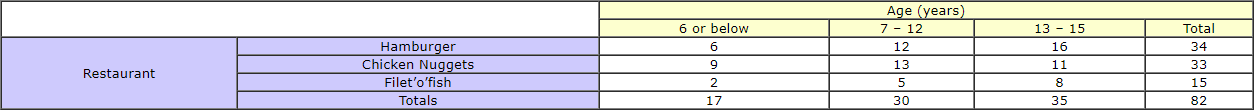A

13/82

B

28/82

C

33/82

D

13/61

Probability & Descriptive Statistics Q15

The following table classified eighty-two children by age and favorite meals at Mac Donald restaurant. Assume a child is randomly selected. Find the probability of being “13-15” years old OR preferring “Filet’o’fish”.A

8/82

B

50/82

C

8/51

D

42/82

Probability & Descriptive Statistics Q16

The following table classified eighty-two children by age and favorite meals at Mac Donald restaurant. Assume a child is randomly selected. Find the probability of “preferring Hamburger” given that the randomly selected child is 13-15 years old.A

16/82

B

16/35

C

16/34

D

16/70

Probability & Descriptive Statistics Q17

The following table classified eighty-two children by age and favorite meals at Mac Donald restaurant. Assume a child is randomly selected. The events “preferring Hamburger” and “being 13-15 years old” are:A

Mutually exclusive

B

Independent

C

Neither A nor B

D

Both A & B

Probability & Descriptive Statistics Q18

E and F are two events such P(E) = 0.60, P(E or F) = 0.90 and P(E and F) = 0.50. Find P(F).

A

0.80

B

0.30

C

0.40

D

0.10

Probability & Descriptive Statistics Q19

The probability that a randomly chosen adult resident of Bayview city owns a boat is 0.16. The probability that a randomly chosen adult rents an apartment is 0.30. The probability that the adult owns a boat given he/she rents an apartment is 0.20. Find the probability that a randomly chosen adult rents an apartment and owns a boat is:

A

0.048

B

0.24

C

0.10

D

0.06

Probability & Descriptive Statistics Q20

Possessing a boat and renting an apartment are:

A

independent events

B

mutually exclusive

C

both independent and mutually exclusive

D

neither independent nor mutually exclusive

Probability & Descriptive Statistics Q21

A bag contains 4 red marbles and 5 blue marbles. Two marbles are randomly drawn without replacement. Find the probability of the event “The first marble is red and the second is blue.”

A

20/81

B

20/72

C

4/12

D

4/9

Probability & Descriptive Statistics Q22

A bag contains 4 red marbles and 5 blue marbles. Two marbles are randomly drawn without replacement. Find the probability that both marbles are red.

A

16/81

B

7/81

C

12/ 72

D

8/72

Probability & Descriptive Statistics Q23

Approximately 70% of U. S. adults had at least one pet as a child. We randomly survey 60 U.S. adults. We are interested in the number that had at least one pet as a child. The probability that at least 3 adults had at least one pet as a child means:

A

P(X=0)+P(X=1)+P(X=2)

B

P(X=0)+P(X=1)+P(X=2)+P(X=3)

C

P(X=4)+P(X=5)+P(X=6)+ ... + P(X = 60)

D

P(X=3)+P(X=4)+P(X=5)+ ... + P(X = 60)

Probability & Descriptive Statistics Q24

A plumber has determined the possible number of house calls to be made each day, and their related probabilities. What is the probability that he makes at least 1, but no more than 3 house calls in a day?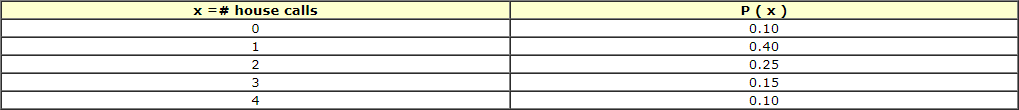A

0.65

B

0.80

C

0.50

D

0.40

Probability & Descriptive Statistics Q25

A plumber has determined the possible number of house calls to be made each day, and their related probabilities. If the plumber charges a flat fee of $40 for a house call, the expected daily income is:A$70

B

$175 C$400

D

\$1.75

Probability & Descriptive Statistics Q26

If X ~ B ( 40 , 0.2 ) , then P ( X > 11 ) =

A

0.0432

B

0.0001

C

0.0875

D

0.1608

Probability & Descriptive Statistics Q27

The Fizz–Full Soda Company knows that 4% of the bottles of soda it produces are filled with less soda than required. If one purchases 10 bottles at random, the probability that at most 2 of these bottles will have less soda than required is:

A

0.0519

B

0.9938

C

0.9418

D

0.0080

Probability & Descriptive Statistics Q28

Assume the statistics final is a multiple-choice exam with 50 questions, each question having 5 choices, only one of which is correct. Assume you answer all questions at random (guessing). The expected number of questions you would get correct would be:

A

5

B

10

C

40

D

45

Probability & Descriptive Statistics Q29

Assume the statistics final is a multiple-choice exam with 50 questions, each question having 5 choices, only one of which is correct. Assume you answer all questions at random (guessing).Based upon numerical calculations, would you be surprised if a person got exactly half of the questions correct?

A

yes, because it is impossible

B

yes, because the probability is almost 0

C

no, because the probability is 0.50

D

no, because it is the most likely probability

Probability & Descriptive Statistics Q30

If sampling without replacement occurs, do the picks follow the Binomial Distribution?

A

Yes, because each pick is independent from the others.

B

No, because the probability of success on each pick changes.

C

Yes, if we are counting the number of successes.

D

No, because we may not have any successes.

Probability & Descriptive Statistics Q31

Ninety-four percent of California community college transfers feel that their community college adequately prepared them to handle upper-division coursework at their transfer university. We randomly survey 14 California community college transfers. We are interested in the number that feel that their community college adequately prepared them to handle upper division coursework at their transfer university. List the values that X, the Random Variable, may take on.

A

1, 2, 3, ..., 14

B

1, 2, 3, ..., 94

C

0, 1, 2, ..., 14

D

0, 1, 2, ..., 94

CC BY - Probability & Descriptive Statistics

Content Developed by Susan Dean and Barbara Illowsky, Licensed under a CC BY-NC 1.0

Published by the Sofia Open Content Initiative

© 2004 Foothill-De Anza Community College District &The William and Flora Hewlett Foundation